# Create BMI (Body Mass Index) Calculator In Java

In this tutorial you will learn about the Create BMI (Body Mass Index) Calculator In Java and its application with practical example.

In this tutorial, we will learn to create a Create BMI (Body Mass Index) Calculator In Java using Java programming.

## Prerequisites

Before starting with this tutorial we assume that you are best aware of the following Java programming topics:

• Java Operators.
• Basic Input and Output function in Java.
• Class and Object in Java.
• Basic Java programming. Basic Java programming.
• If-else statements in Java.
• For loop in Java.

## What is Body Mass Index (BMI)

It is measured on the basis of “health,height and weight”. So it can be calculated by taking the weight in kg and by dividing the square of your height in meters.

Formula of Calculating BMI.

BMI = (Weight in Kg) / (Height in Meters * Height in Meters).

Example
weight = 70 kg, height = 1.2 m
BMI = 70 / (1.65*1.65) = BMI= 25.7 kg/m2

• Healthy BMI range is :=> 18.5 kg/m2 – 25 kg/m2
• Healthy weight for the height ranges :=> 50.4 kgs – 68.1 kgs
• You need to Lose 1.9 kgs to reach a BMI of 25 kg/m2.
 BMI Range Category BMI   < 18.5 Thinness BMI 18.5 – 25 Normal BMI   25 – 30 Overweight BMI> 30 Obese

## Create BMI (Body Mass Index) Calculator In Java

In this program we will find BMI(Body Mass Index). We would first declared and initialized the required variables. Next, we would prompt user to input height and weight.Later we will find the BMI. Let’s have a look at the program.

## Output

BMI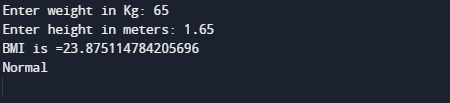In the above program, we have first declared and initialized a set variables required in the program.

• weight =It will hold value of kg.
• meter =It will hold value in meter.
• BMI =  For calculating BMI.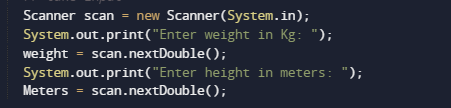After that taking value of weight in kg and height in meter we pass these values to function bmicalculator (weight,height).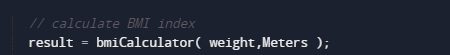After taking values from user bmicalculator () method takes two arguments and calculate bmi as shown in image below.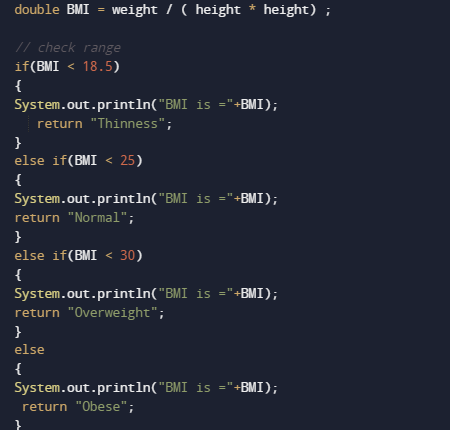Here BMI calculator finds that whether the person is underweight, normal, overweight, or obese and the print result.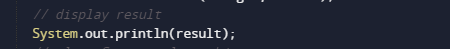In this tutorial we have learn about the Create BMI (Body Mass Index) Calculator In Java and its application with practical example. I hope you will like this tutorial.